# 1. 哲学上先验的概念

## 0x1：人类知性的组成

1. 一是逻辑功能：逻辑功能在知识的基础上规定着思维的判断形式，这是形式逻辑的范围；
2. 一是认识功能：认识功能则为我们提供新的知识，这是“先验逻辑”的领域；

## 0x2：康德”哥白尼式的革命“

### 2. 认识的来源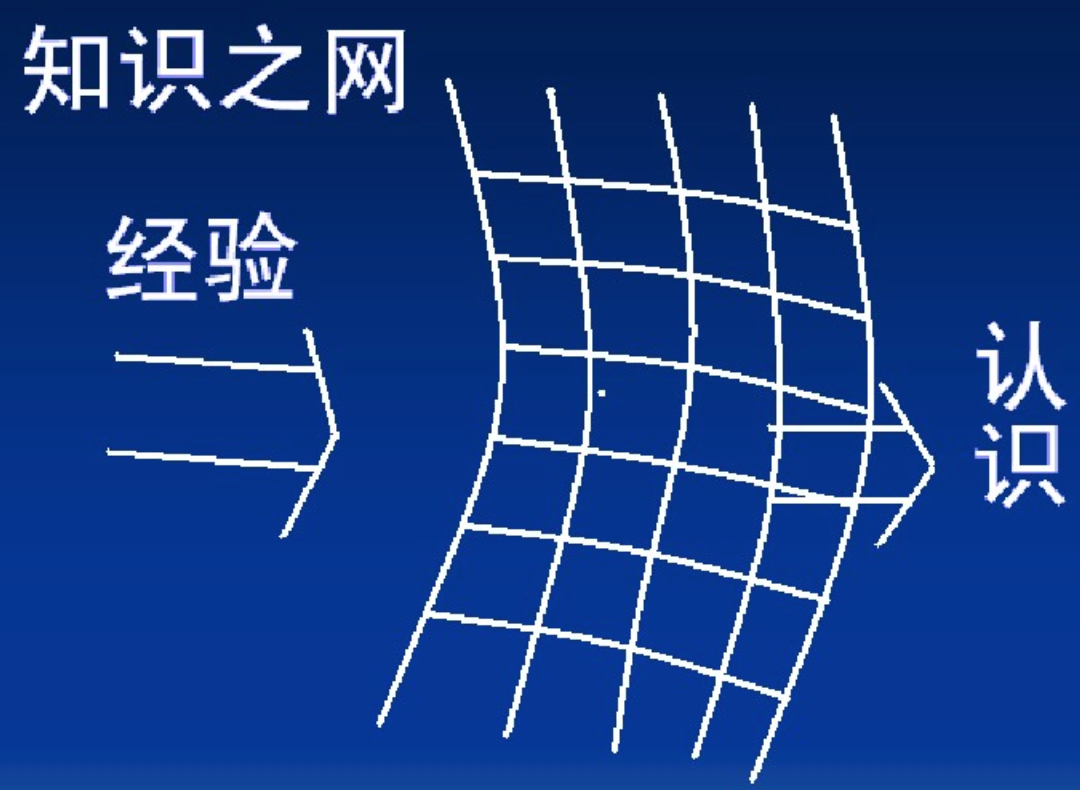### 4. 先验调和了理性主义和经验主义

https://baike.baidu.com/item/%E5%85%88%E9%AA%8C%E5%88%86%E6%9E%90%E5%88%A4%E6%96%AD/7101736
https://wenku.baidu.com/view/18cce387e53a580216fcfedd.html
《实践理性批判》康德

# 2. 主观和客观先验

1. 客观先验：皆在让数据最大程度地影响后验；
2. 主观先验：让领域专家来表达自己对先验的个人看法；

## 0x1：客观先验

### 2. 杰弗里斯先验 - 建立一个不会因偶然改变变量位置而大幅变化的先验

# -*- coding: utf-8 -*-

import numpy as np
from IPython.core.pylabtools import figsize
import matplotlib.pyplot as plt
import scipy.stats as stats

x = np.linspace(0.000, 1, 150)
y = np.linspace(1.0, 1.0, 150)
lines = plt.plot(x, y, color="#A60628", lw=3)
plt.fill_between(x, 0, y, alpha=0.2, color=lines.get_color())
plt.autoscale(tight=True)
plt.ylim(0, 2)

plt.show()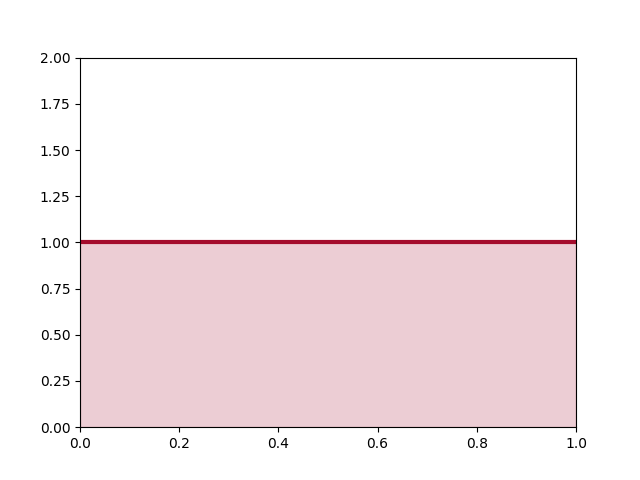# -*- coding: utf-8 -*-

import numpy as np
from IPython.core.pylabtools import figsize
import matplotlib.pyplot as plt
import scipy.stats as stats

psi = np.linspace(-10, 10, 150)
y = np.exp(psi) / (1 + np.exp(psi)) ** 2
lines = plt.plot(psi, y, color="#A60628", lw=3)
plt.fill_between(psi, 0, y, alpha=0.2, color=lines.get_color())
plt.autoscale(tight=True)
plt.ylim(0, 1)

plt.show()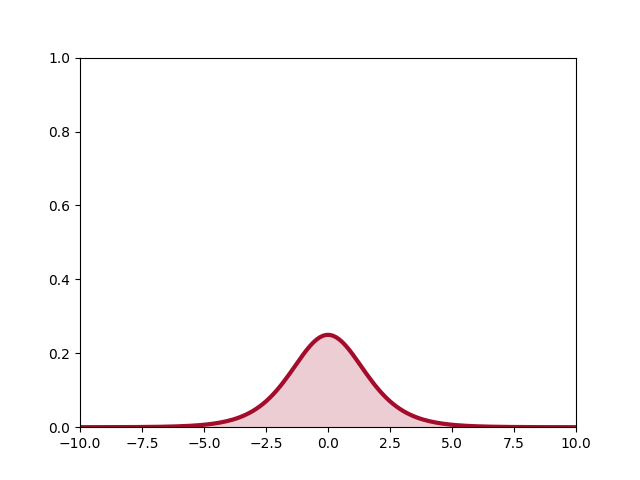## 0x2：主观先验

### 1. 对参数空间中特定区域有主观偏倚的先验分布

# -*- coding: utf-8 -*-

import numpy as np
from IPython.core.pylabtools import figsize
import matplotlib.pyplot as plt
import scipy.stats as stats

colors = ["#348ABD", "#A60628", "#7A68A6", "#467821"]

x = np.linspace(0, 1)
y1, y2 = stats.beta.pdf(x, 1, 1), stats.beta.pdf(x, 10, 10)

p = plt.plot(x, y1,
label='An objective prior \n(uninformative, \n"Principle of Indifference" )')
plt.fill_between(x, 0, y1, color=p.get_color(), alpha=0.3)

p = plt.plot(x, y2,
label="A subjective prior \n(informative)")
plt.fill_between(x, 0, y2, color=p.get_color(), alpha=0.3)

p = plt.plot(x[25:], 2 * np.ones(25), label="another subjective prior")
plt.fill_between(x[25:], 0, 2, color=p.get_color(), alpha=0.3)

plt.ylim(0, 4)

plt.ylim(0, 4)
leg = plt.legend(loc="upper left")
leg.get_frame().set_alpha(0.4)
plt.title("Comparing objective vs. subjective priors for an unknown probability")

plt.show()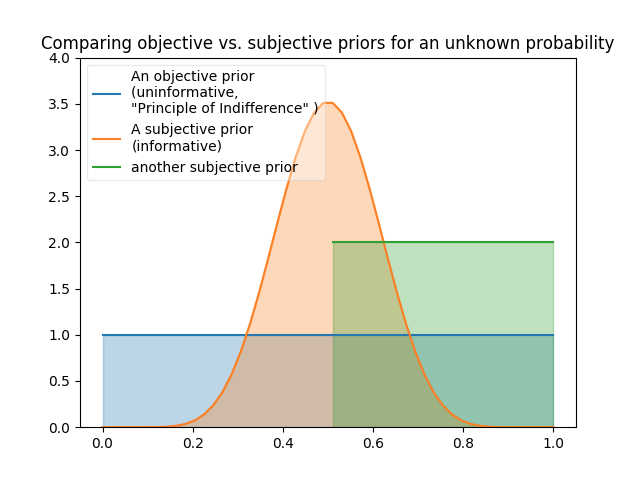### 3. 从领域专家处获得先验分布

1. 有助于MCMC收敛：例如如果我们知道未知参数是严格为正的，那么我们可以缩小关注空间，从而省下在负值查找的时间；
2. 允许更准确的推断：通过加权靠近真值的先验，我们收窄我们的最终推断；
3. 更好地表达了我们的不确定性；

## 0x4：经验贝叶斯 - 基于数据驱动的先验选择

### 1. 一个简单的例子 - 估计模型超参数

1. 经验贝叶斯：使用经验的样本均值，这将使先验居中于经验样本均值：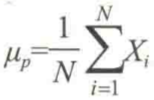2. 传统贝叶斯推断：使用主观先验知识，或者更客观的先验（0均值和大的标准差）

https://www.zhihu.com/question/67846877/answer/257216646
《思考：快与慢》丹尼尔·卡尼曼 - 锚定相关主题

# 3. 先验的表现形式

1. 在模型设计中引入正则化技术：从贝叶斯的角度来看，正则化等价于对模型参数引入先验分布。
2. 在特征工程中结合领域经验设计特殊的特征向量：例如设计【身高、体重、握力、百米跑步速度】这几位特征维度用于检测学校男生还是女生，显然样本集在这种特征空间上是明显可分的。在特征工程的阶段就得到一个明显可分的样本集是非常值得高兴的，因为这意味着我们的项目已经成功了90%，接下来的工作即使用随机森林可能也能得到非常好的分类结果。
3. 在贝叶斯推断中使用特定先验概率分布函数
4. 模型参数初始化时人工设定特定的初始化值

# 4. 贝叶斯分析中一些常用的先验

1. 思考gamma函数这类函数被发明的最早的原因，是因为其可以作为某个物理现象或当时的实际问题的数学模型；
2. 理论上，任意一个非负的可积函数，你都可以通过变换变成某个分布的密度函数；
3. 数学领域有句名言，数学是解开宇宙秘密的一把钥匙，很多数学公式，你第一眼看到就会从心底产生一个感觉，哇！好美啊！好工整好对称啊！这类数学模型往往可以抽象概括一大类物理现象，因此很自然地被作为先验模型使用。

## 0x1：二项分布 - N次二项实验（只有两种互斥结果的实验）中的离散概率密度函数分布

### 1. 数学公式 - N次实验中发生K次事件的”累计“概率

N次独立重复试验中发生k次的概率是：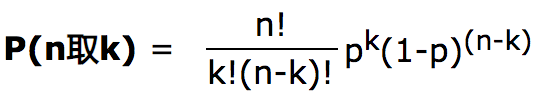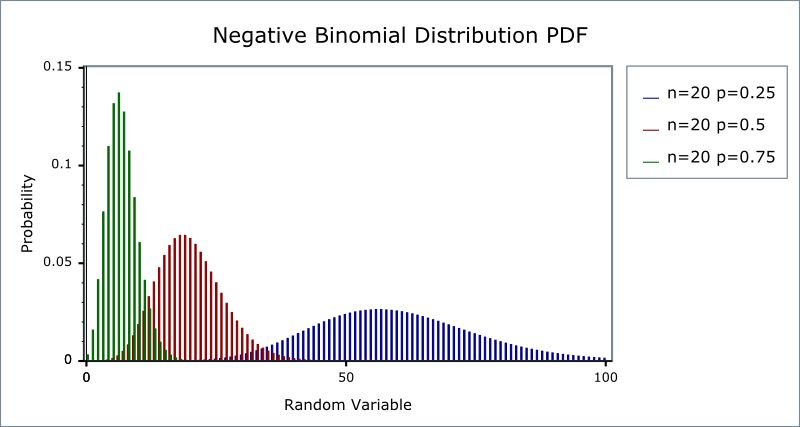### 2. 公式的原理推导和理解

#### 1）等概率伯努利实验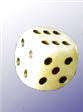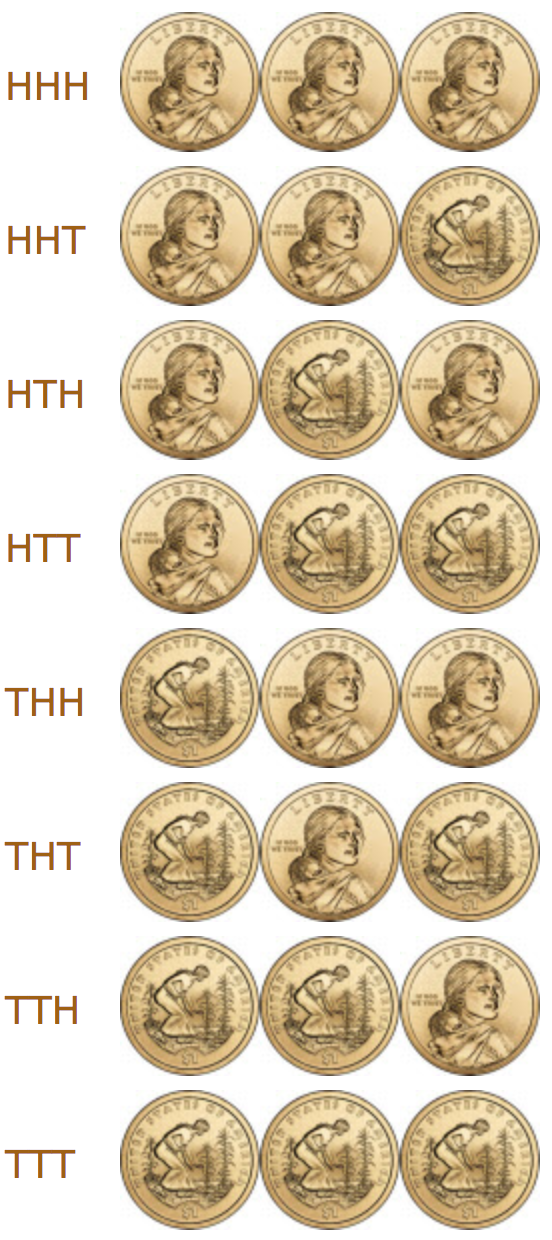"两个正面" 可以是任何次序： "HHT"、"THH" 和 "HTH" 都有两个正面（和一个反面）。所以3个结果有 "两个正面"。

• P(三个正面) = P(HHH) = 1/8
• P(两个正面) = P(HHT) + P(HTH) + P(THH) = 1/8 + 1/8 + 1/8 = 3/8
• P(一个正面) = P(HTT) + P(THT) + P(TTH) = 1/8 + 1/8 + 1/8 = 3/8
• P(没有正面) = P(TTT) = 1/8

将概率分布画在直方图上：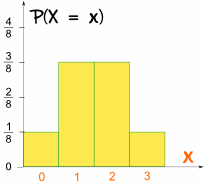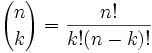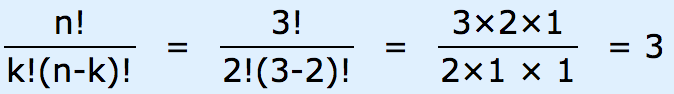#### 2）非等概率伯努利实验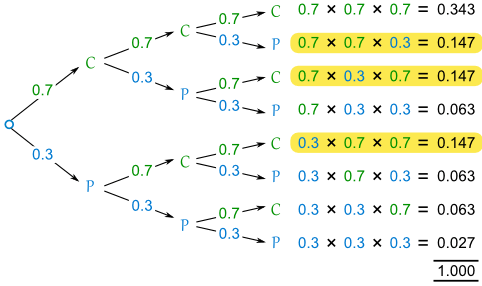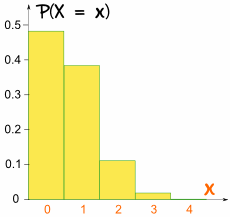0.7 是正面的概率，称它为 p；

2 是目标的结果个数，称它为 k；

"其他的结果" 的概率是：(1-p)(n-k)；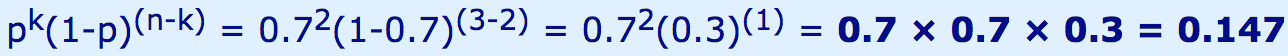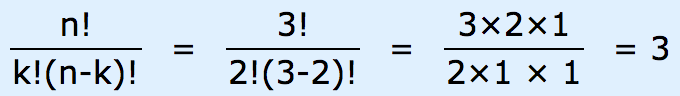### 3. 应用条件

1．各观察单位只能具有相互对立的一种结果，如阳性或阴性，生存或死亡等，属于两分类资料；
2．已知发生某一结果（阳性）的概率为π，其对立结果的概率为1-π，实际工作中要求π是从大量观察中获得比较稳定的数值；
3．n次试验在相同条件下进行，且各个观察单位的观察结果相互独立，即每个观察单位的观察结果不会影响到其他观察单位的结果。如要求疾病无传染性、无家族性等；

https://www.shuxuele.com/data/binomial-distribution.html

## 0x2：泊松分布 - n趋向无穷时的离散二项分布

Poisson分布，是一种离散概率分布，由法国数学家西莫恩·德尼·泊松（Siméon-Denis Poisson）在1838年时发表。

### 1. 数学公式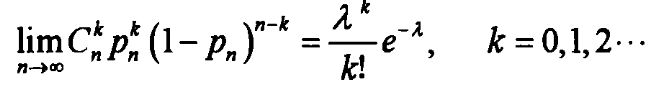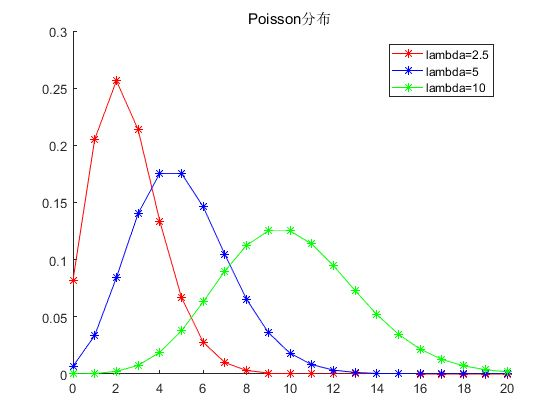### 2. 公式的原理推导和理解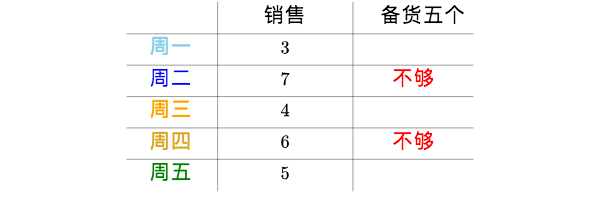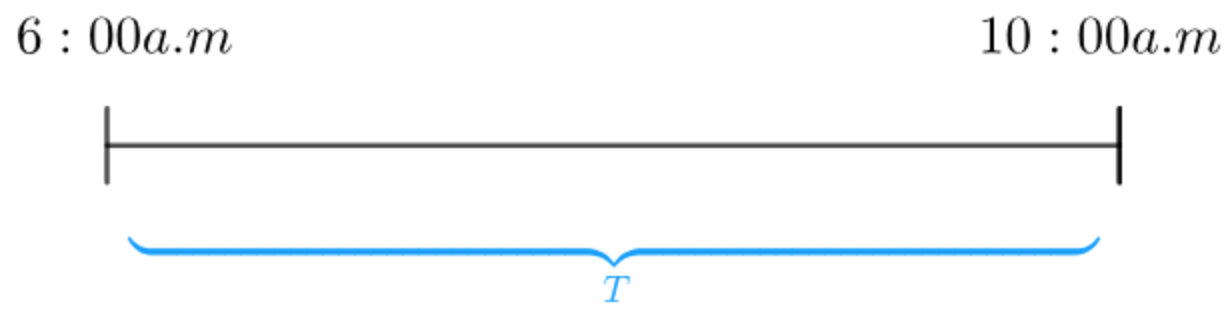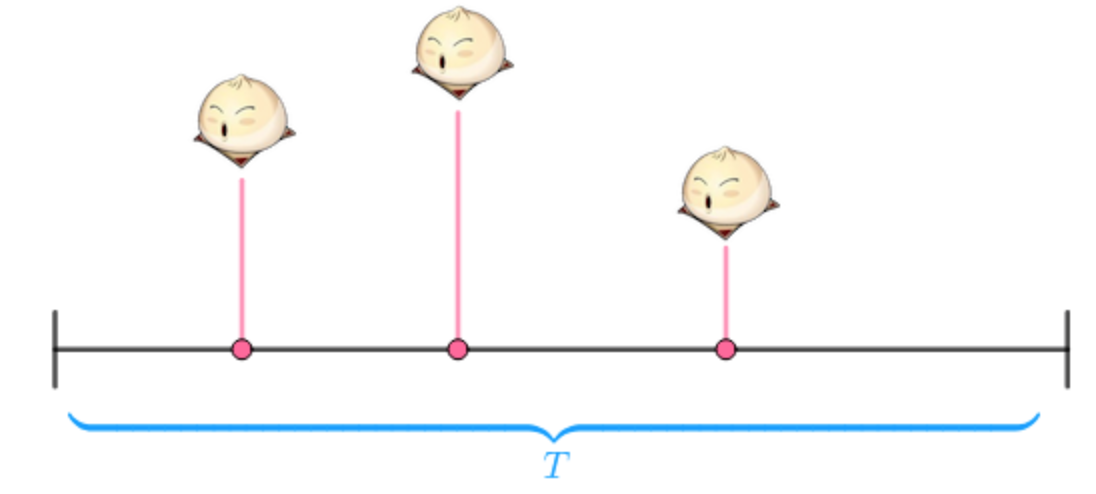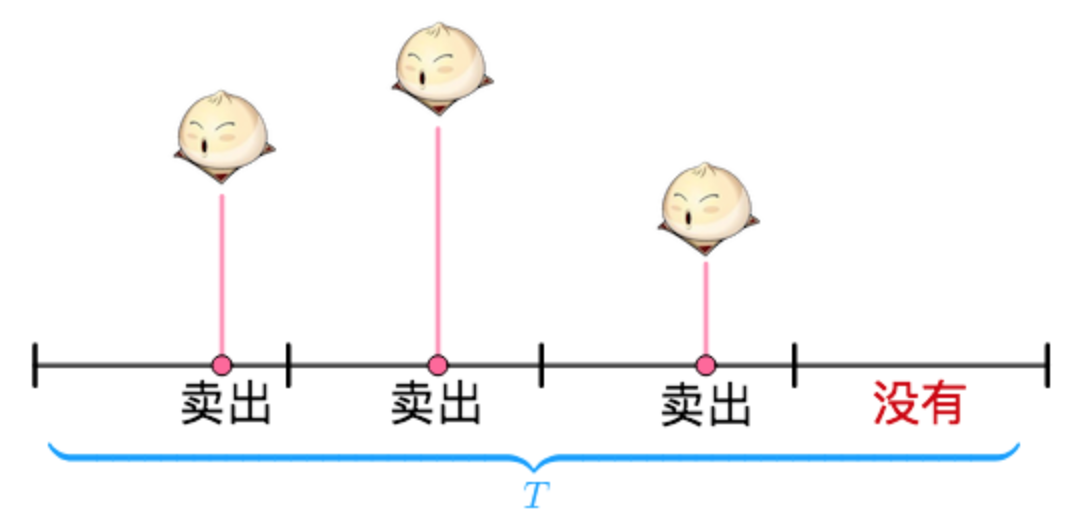1. 每次试验是独立的：T内的每个时间段是否卖出馒头都是条件独立的；
2. 每个试验只有两个可能结果：卖出 or 不卖出；
3. 每个试验里的 "成功" 概率是不变的：我们假定顾客购买这个行为是固定概率的；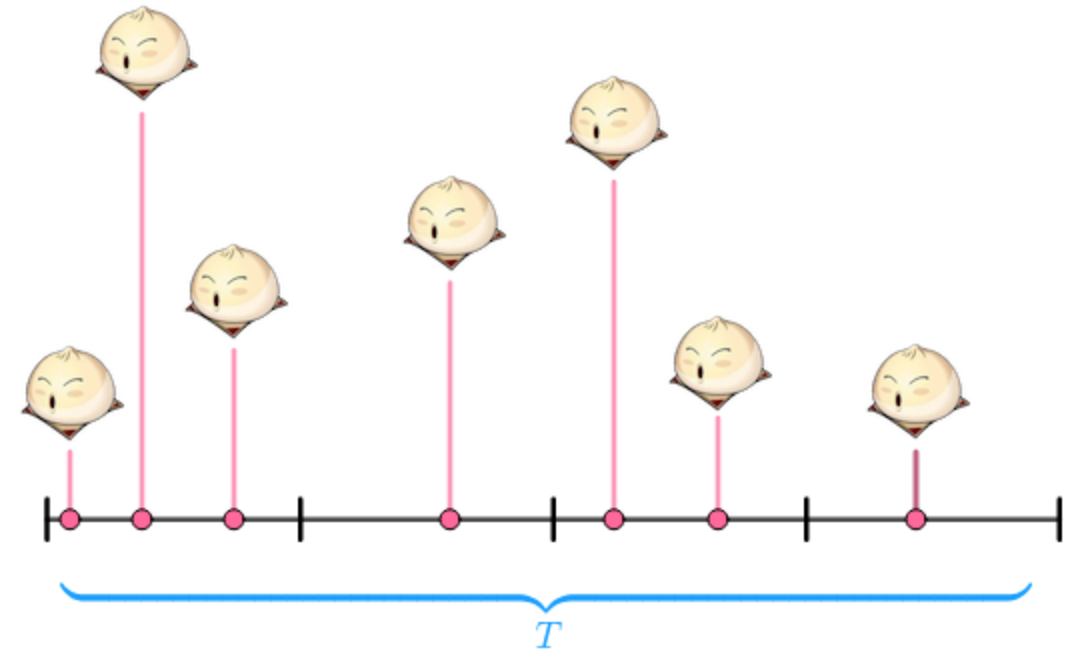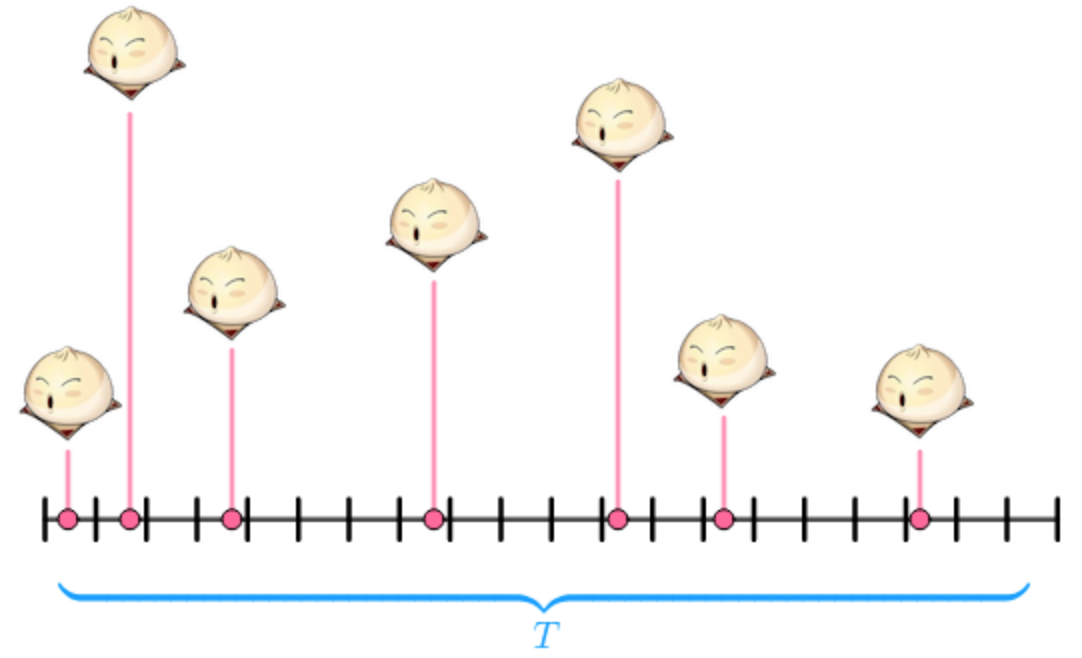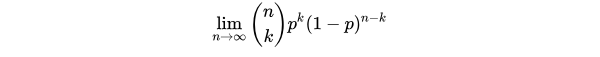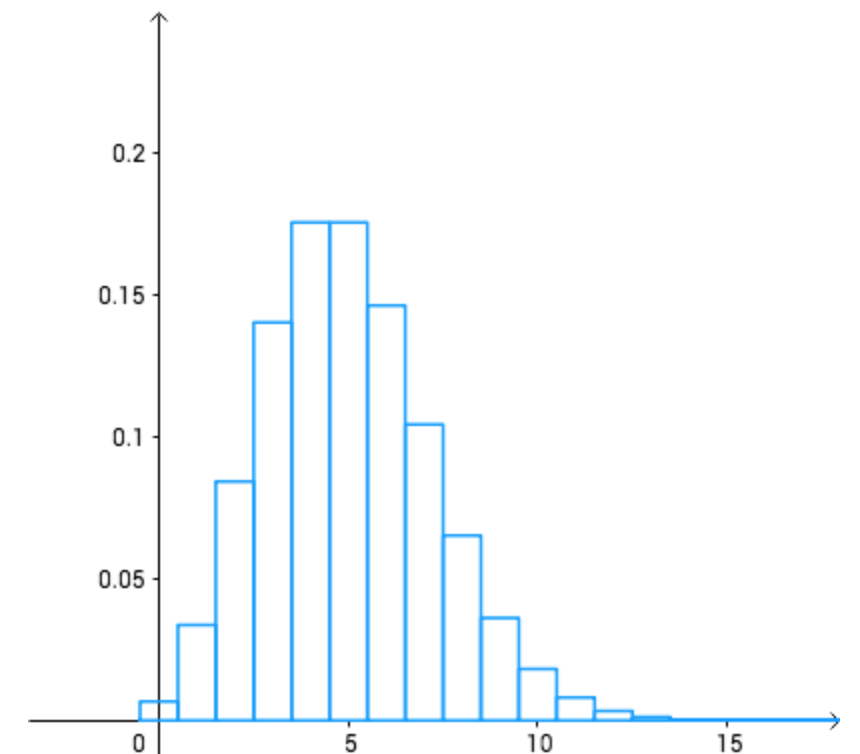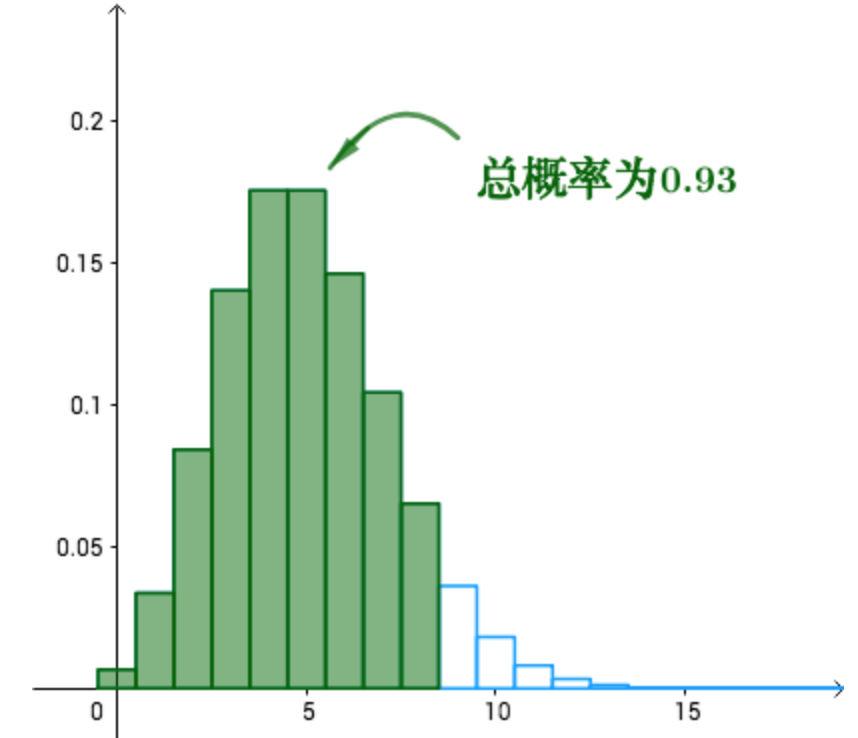### 3. 物理意义

https://blog.csdn.net/ccnt_2012/article/details/81114920
https://blog.csdn.net/ccnt_2012/article/details/81114920 

## 0x3：正态分布 - 连续概率密度分布

### 1. 数学公式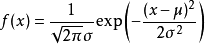### 2. 图形特征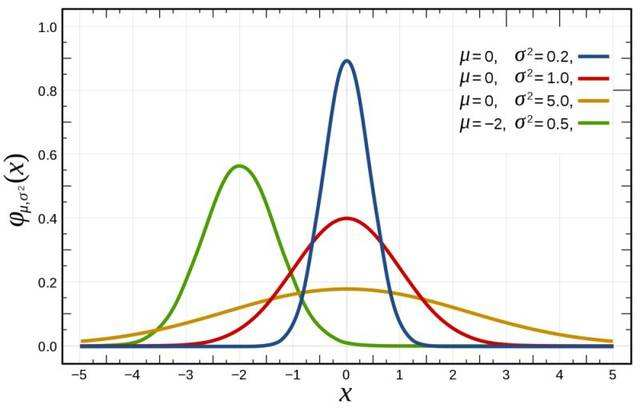1. 集中性：正态曲线的高峰位于正中央，即均数所在的位置。
2. 对称性：正态曲线以均数为中心，左右对称，曲线两端永远不与横轴相交。
3. 均匀变动性：正态曲线由均数所在处开始，分别向左右两侧逐渐均匀下降。

### 3. 物理意义

https://www.zhihu.com/question/26854682
https://baike.baidu.com/item/%E6%AD%A3%E6%80%81%E5%88%86%E5%B8%83/829892?fr=aladdin

## 0x4：Gamma（伽马）分布- 连续概率函数

### 1. 数学公式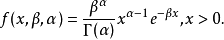Gamma分布中的参数α称为形状参数（shape parameter），β称为逆尺度参数（scale parameter）

### 2. 变化趋势

# -*- coding: utf-8 -*-

import numpy as np
from IPython.core.pylabtools import figsize
import matplotlib.pyplot as plt
import scipy.stats as stats

figsize(12.5, 5)
gamma = stats.gamma

parameters = [(1, 0.5), (9, 2), (3, 0.5), (7, 0.5)]
x = np.linspace(0.001, 20, 150)
for alpha, beta in parameters:
y = gamma.pdf(x, alpha, scale=1. / beta)
lines = plt.plot(x, y, label="(%.1f,%.1f)" % (alpha, beta), lw=3)
plt.fill_between(x, 0, y, alpha=0.2, color=lines.get_color())
plt.autoscale(tight=True)

plt.legend(title=r"$\alpha, \beta$ - parameters")

plt.show()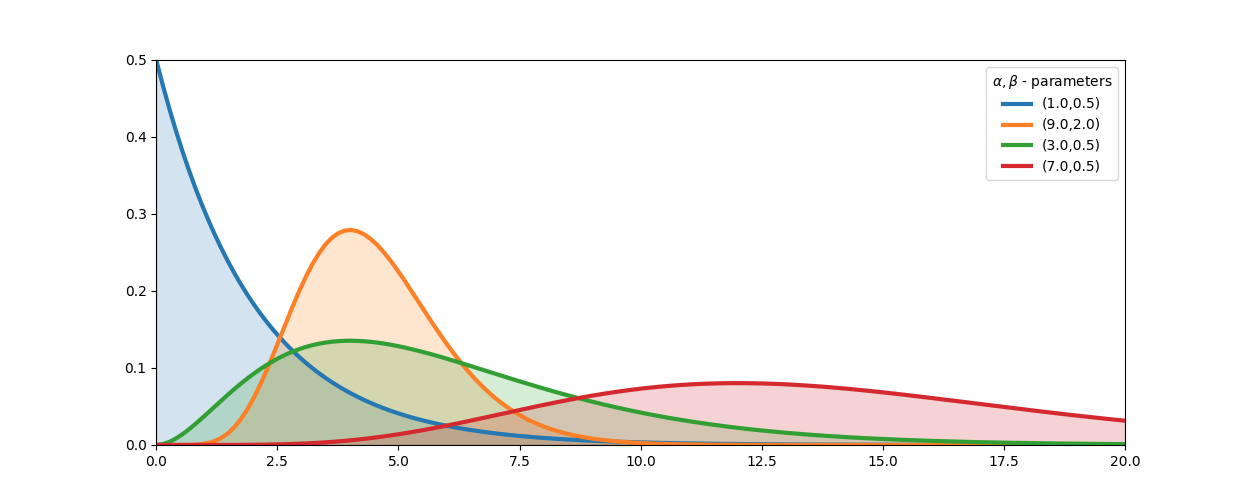https://www.cnblogs.com/coshaho/p/9653460.html
https://www.cnblogs.com/alps/p/5601300.html
https://www.zhihu.com/question/34866983/answer/60541847

## 0x5：威沙特分布

# -*- coding: utf-8 -*-

import numpy as np
from IPython.core.pylabtools import figsize
import matplotlib.pyplot as plt
import scipy.stats as stats

figsize(12.5, 5)

n = 4
for i in range(10):
ax = plt.subplot(2, 5, i + 1)
if i >= 5:
n = 15
plt.imshow(stats.wishart.rvs(n + 1, np.eye(n)), interpolation="none",
cmap="hot")
ax.axis("off")

plt.suptitle("Random matrices from a Wishart Distribution")

plt.show()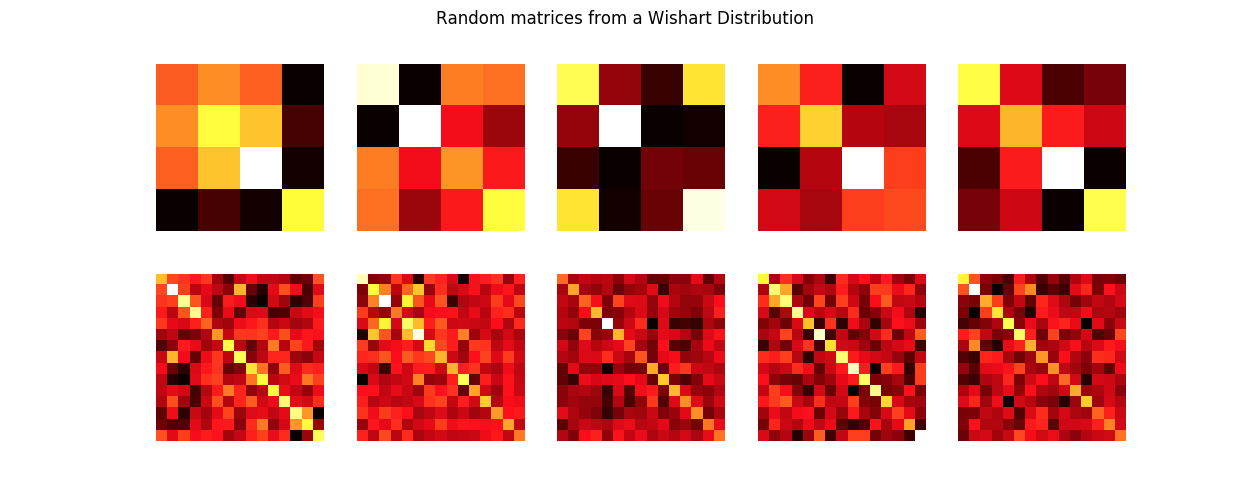## 0x6：Beta分布

### 1. 数学公式

Β分布的概率密度函数是：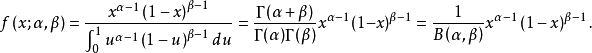### 2. 变化趋势

α，β参数决定了Beta分布的形状：

# -*- coding: utf-8 -*-

import numpy as np
from IPython.core.pylabtools import figsize
import matplotlib.pyplot as plt
import scipy.stats as stats

figsize(12.5, 5)

params = [(2, 5), (1, 1), (0.5, 0.5), (5, 5), (20, 4), (5, 1)]

x = np.linspace(0.01, .99, 100)
beta = stats.beta
for a, b in params:
y = beta.pdf(x, a, b)
lines = plt.plot(x, y, label = "(%.1f,%.1f)"%(a,b), lw = 3)
plt.fill_between(x, 0, y, alpha = 0.2, color = lines.get_color())
plt.autoscale(tight=True)
plt.ylim(0)
plt.legend(loc = 'upper left', title="(a,b)-parameters")

plt.show()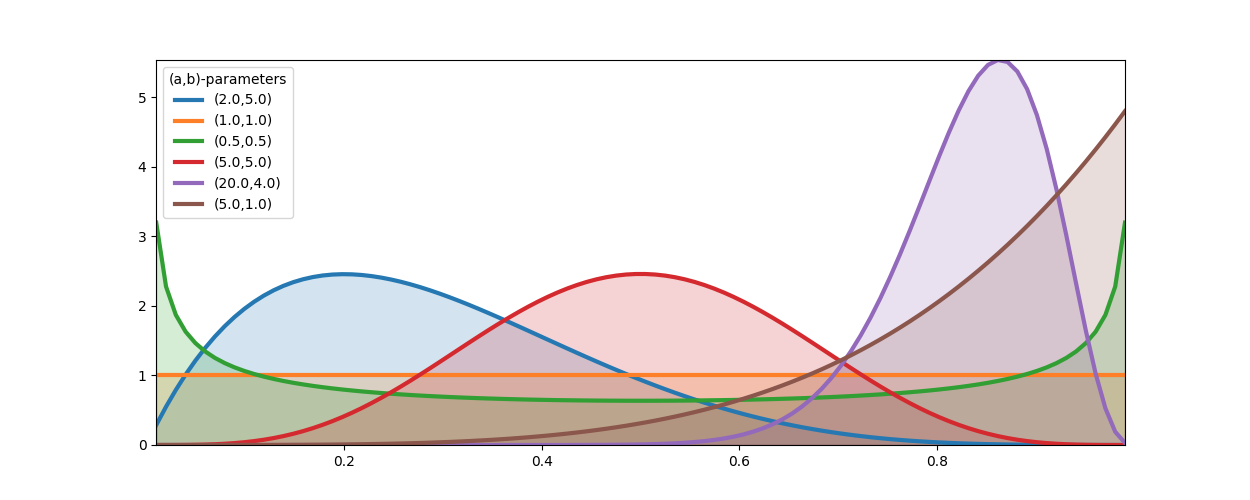### 3. Beta分布特性

1. 作为概率的概率分布，Beta(a, b)在(0, 1)上对 θ 积分必定为1；
2. Beta(a, b)同时能作为先验分布和后验分布，能够模拟各种概率分布情况；
3. Beta分布可以模拟出以(0, 1)上任意点为峰值的曲线，这表明Beta分布可以模拟极大似然法求出的任意最大值点概率值；

https://baike.baidu.com/item/%E8%B4%9D%E5%A1%94%E5%88%86%E5%B8%83/8994021?fr=aladdin
http://www.cnblogs.com/coshaho/p/9658135.html

### 1. 什么是共轭先验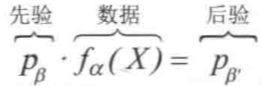### 2. 共轭先验的性质

1. 代数上的方便性，可以在建模时直接给出后验分布的封闭形式，否则的话只能数值计算（例如SGD启发式搜索）。
2. 可以形成一个先验链，即现在的后验分布可以作为下一次计算的先验分布，如果形式相同，就可以形成一个链条。
3. 一切指数族分布成员都存在一个共轭先验。
1）在伯努利分布中，共轭先验是 Beta 分布；
2）在高斯分布中，均值的共轭先验是高斯分布，精度的共轭先验是Wishart分布；

### 3. 共轭先验的局限

1. 共轭先验是不客观的，因此，它只有当需要主观先验时才有用。不能保证共轭先验能够顾忌开发者的主观意识；
2. 对于简单的一维问题，通常存在共轭先验。但是对于更大更复杂的问题，涉及更复杂的结构，基本没希望找到共轭先验；

### 4. 一个例子：Beta先验+二项分布数据 = Beta后验分布

Beta分布和二项分布之间有一个有趣的关系。假设我们感兴趣的是一些未知的比例或者概率 p。我们设定它符合一个Beta(α，β) 先验分布，然后我们通过观察收集一个由二项式过程 X~B(N，p) 昌盛的数据，其中 p 仍然是未知的，后验分布仍然是Beta分布，即：p | X ~ Beta（a+X，β+N-X）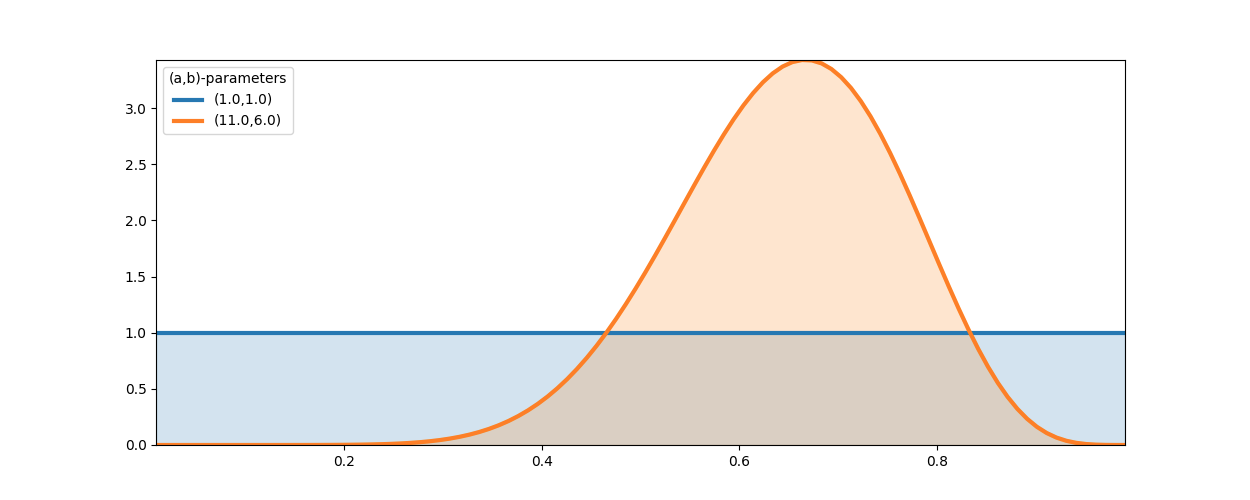https://www.jianshu.com/p/8de4fd24e53c

## 0x8：各个分布之间的推演和转化关系：

### 1. 二项分布和泊松分布的关系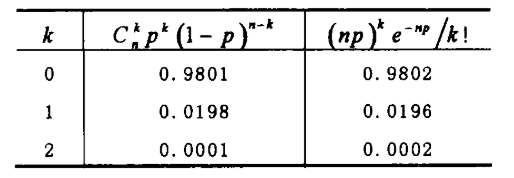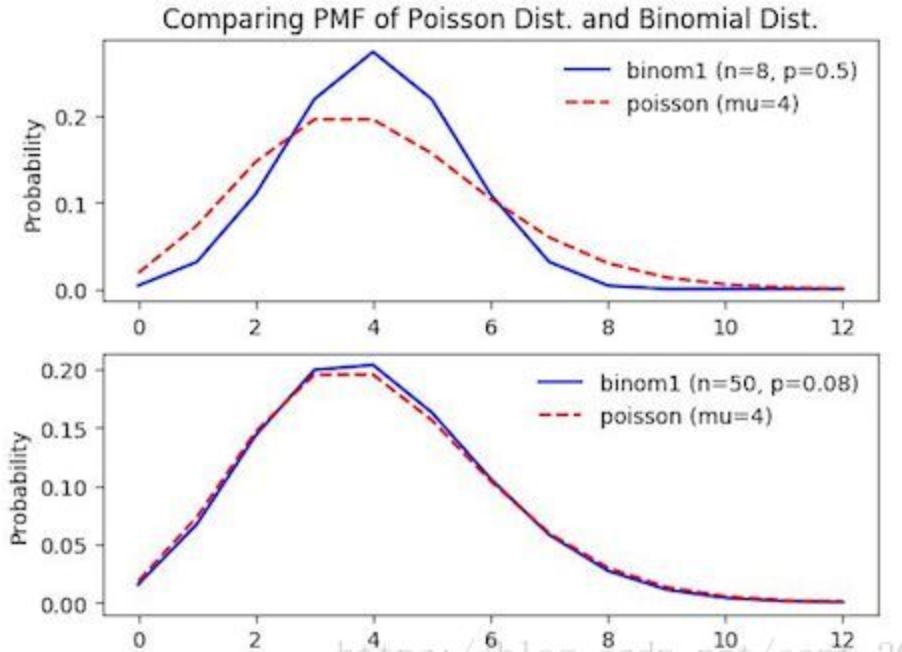### 2. 二项分布和正态分布的关系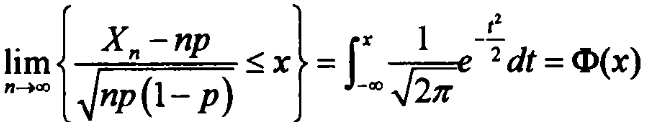### 3. 泊松分布和正态分布的关系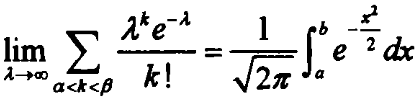，其中，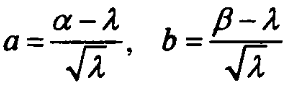### 4. 用泊松分布还是正态分布来近似二项分布？

1. 当 p 很小时，即使 n 不是很大，用泊松分布近似二项分布，已经相当吻合。但是在这种情况下，如果用正态分布来近似就会造成较大的误差；
2. 当 n 充分大，p 既不接近于0也不接近于1时（最好满足 0.1<=p<0.9），正态分布可以较好地近似二项分布；

https://wenku.baidu.com/view/5d9ac306e87101f69e3195e4.html
https://www.zybang.com/question/dcdea243cfc493eac4dde9eb4c05b373.html
https://baike.baidu.com/item/Gamma%E5%88%86%E5%B8%83/1033808
http://www.cnblogs.com/JustForCS/p/5264315.html
https://blog.csdn.net/u012279165/article/details/73693157
http://www.360doc.com/content/17/1231/22/9200790_718001949.shtml 

# 5. 数据量N和先验分布对最终后验的综合决策

## 0x1：通过乘积公式体现数据量和先验分布对后验估计的动态制衡作用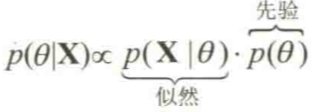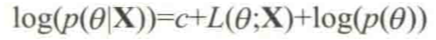## 0x2：选择退化的先验

# -*- coding: utf-8 -*-

import numpy as np
from IPython.core.pylabtools import figsize
import matplotlib.pyplot as plt
import scipy.stats as stats
import pymc as pm

figsize(12.5, 15)

p_actual = 0.35
x = np.random.binomial(1, p_actual, size=100)
print x[:10]

p = pm.Uniform('p', 0.5, 1)
obs = pm.Bernoulli('obs', p, value=x, observed=True)

mcmc = pm.MCMC([p, obs])
mcmc.sample(10000, 2000)

p_trace = mcmc.trace('p')[:]
plt.xlabel('Value')
plt.ylabel('Density')
plt.hist(p_trace, bins=30, histtype='stepfilled', normed=True)

plt.show()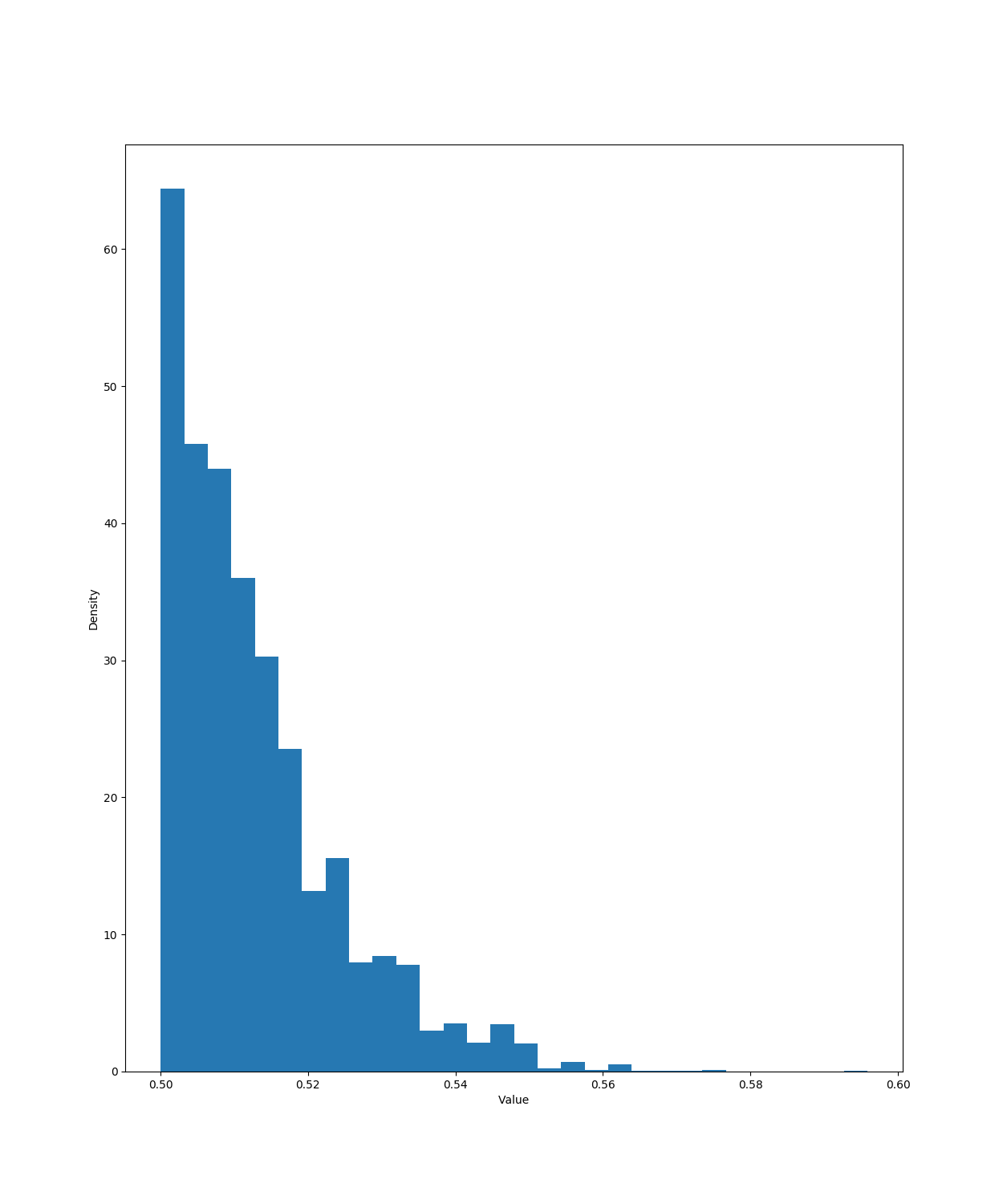## 0x3：一个例子说明数据对先验的修正作用

# -*- coding: utf-8 -*-

import numpy as np
from IPython.core.pylabtools import figsize
import matplotlib.pyplot as plt
import scipy.stats as stats
import pymc as pm

figsize(12.5, 15)

p = 0.6
beta1_params = np.array([1.,1.])
beta2_params = np.array([2,10])
beta = stats.beta

x = np.linspace(0.00, 1, 125)
data = stats.bernoulli.rvs(p, size=500)

plt.figure()
for i,N in enumerate([0,4,8, 32,64, 128, 500]):
s = data[:N].sum()
plt.subplot(8,1,i+1)
params1 = beta1_params + np.array([s, N-s])
params2 = beta2_params + np.array([s, N-s])
y1,y2 = beta.pdf(x, *params1), beta.pdf( x, *params2)
plt.plot(x,y1, label = r"flat prior", lw =3)
plt.plot(x, y2, label = "biased prior", lw= 3)
plt.fill_between(x, 0, y1, color ="#348ABD", alpha = 0.15)
plt.fill_between(x, 0, y2, color ="#A60628", alpha = 0.15)
plt.legend(title = "N=%d" % N)
plt.vlines(p, 0.0, 7.5, linestyles = "--", linewidth=1)
#plt.ylim( 0, 10)#

plt.show()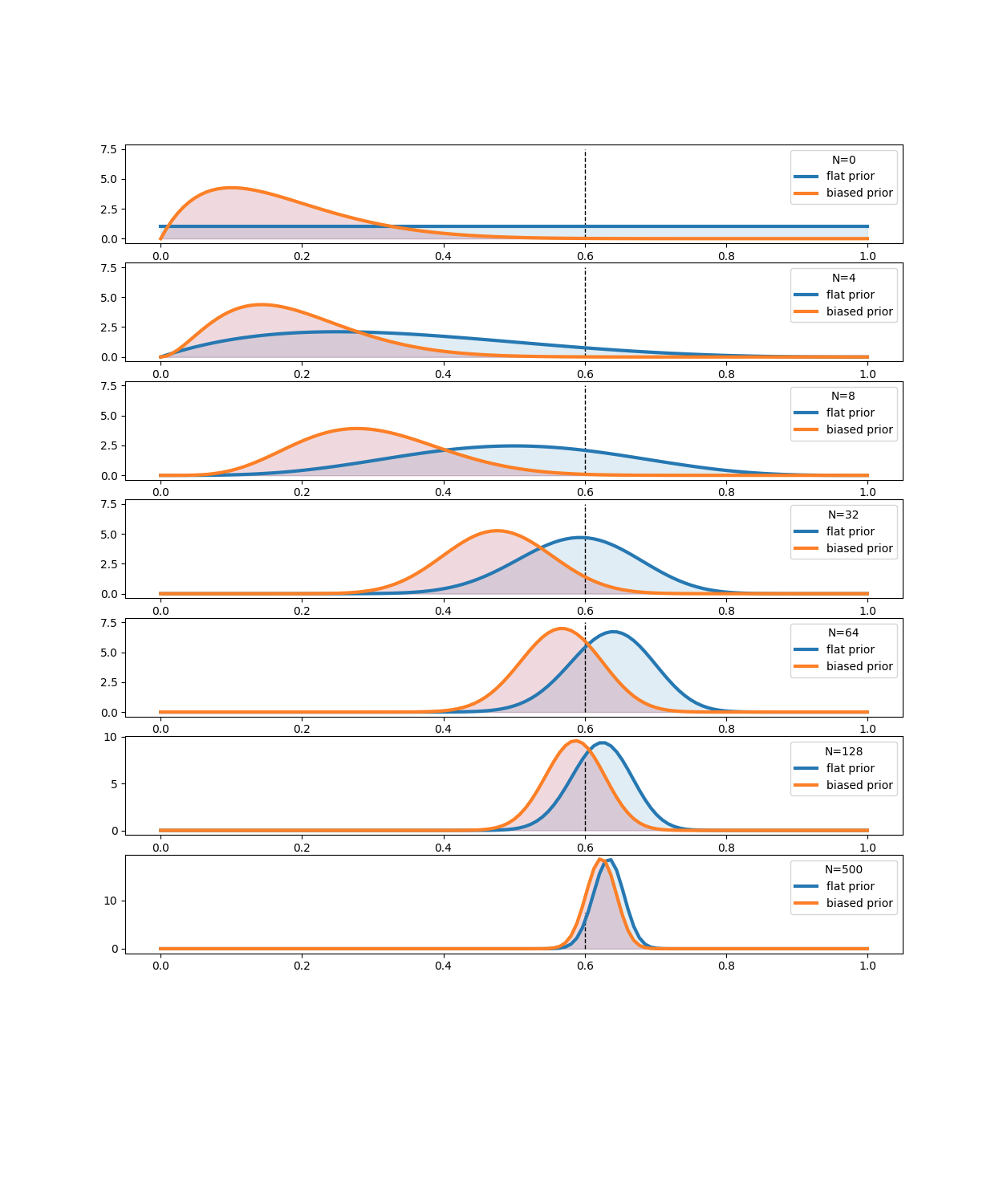《贝叶斯方法》

# 6. 贝叶斯多臂游戏机问题

## 0x2：该问题的现实意义

1. 互联网展示广告：公司有一系列可以展示给潜在客户的广告，但该公司并不清楚要遵循哪些广告策略，以最大限度地提高销售。
2. 生态学：动物只有有限的能量用于耗费，而且某些行为带来的回报是不确定的。动物如何最大化其适应度？
3. 金融：在随时间变化的回报量中，哪些股票期权能给出最高的回报？
4. 临床试验：一位研究人员希望在众多的方案中找出最好的治疗方案，同时最大限度地减少损失。
5. 心理学：赏罚如何影响我们的行为？人类如何学习？

## 0x3：游戏策略

### 2. 算法流程

1. 首轮游戏：对所有游戏机（这里N=10台）设定一个扁平先验，也即初始化阶段是零知识的，对10台游戏机随机进行一次抽取即可；
2. 获取本轮样本数据：选择本轮抽取中，样本值最高的游戏机 b，即选择 B = argmax Xb，根据那个样本值最高的游戏机 b 的样本结果，作为本轮试验的样本数据，【x，y】，x代表第几胎游戏机，y代表对应的值。
3. 更新后验：基于本轮的样本数据【x，y】更新先验分布，这可以理解为一个后验修正过程；
4. 重复2-3过程；

### 3. MCMC推断所有游戏机的发奖率后验分布

# -*- coding: utf-8 -*-

import numpy as np
from IPython.core.pylabtools import figsize
import matplotlib.pyplot as plt
import scipy.stats as stats
import pymc as pm

rand = np.random.rand

class Bandits(object):
"""
This class represents N bandits machines.

parameters:
p_array: a (n,) Numpy array of probabilities >0, <1.

methods:
pull( i ): return the results, 0 or 1, of pulling
the ith bandit.
"""

def __init__(self, p_array):
self.p = p_array
self.optimal = np.argmax(p_array)

def pull(self, i):
# i is which arm to pull
return np.random.rand() < self.p[i]

def __len__(self):
return len(self.p)

class BayesianStrategy(object):
"""
Implements a online, learning strategy to solve
the Multi-Armed Bandit problem.

parameters:
bandits: a Bandit class with .pull method

methods:
sample_bandits(n): sample and train on n pulls.

attributes:
N: the cumulative number of samples
choices: the historical choices as a (N,) array
bb_score: the historical score as a (N,) array
"""

def __init__(self, bandits):
self.bandits = bandits
n_bandits = len(self.bandits)
self.wins = np.zeros(n_bandits)
self.trials = np.zeros(n_bandits)
self.N = 0
self.choices = []
self.bb_score = []

def sample_bandits(self, n=1):
bb_score = np.zeros(n)
choices = np.zeros(n)

for k in range(n):
# sample from the bandits's priors, and select the largest sample
choice = np.argmax(np.random.beta(1 + self.wins, 1 + self.trials - self.wins))

# sample the chosen bandit
result = self.bandits.pull(choice)

# update priors and score
self.wins[choice] += result
self.trials[choice] += 1
bb_score[k] = result
self.N += 1
choices[k] = choice

self.bb_score = np.r_[self.bb_score, bb_score]
self.choices = np.r_[self.choices, choices]
return

figsize(11.0, 10)

beta = stats.beta
x = np.linspace(0.001,.999,200)

def plot_priors(bayesian_strategy, prob, lw = 3, alpha = 0.2, plt_vlines = True):
## plotting function
wins = bayesian_strategy.wins
trials = bayesian_strategy.trials
for i in range(prob.shape):
y = beta(1+wins[i], 1 + trials[i] - wins[i])
p = plt.plot(x, y.pdf(x), lw = lw)
c = p.get_markeredgecolor()
plt.fill_between(x,y.pdf(x),0, color = c, alpha = alpha,
label="underlying probability: %.2f" % prob[i])
if plt_vlines:
plt.vlines(prob[i], 0, y.pdf(prob[i]) ,
colors = c, linestyles = "--", lw = 2)
plt.autoscale(tight = "True")
plt.title("Posteriors After %d pull" % bayesian_strategy.N +\
"s"*(bayesian_strategy.N > 1))
plt.autoscale(tight=True)
return

hidden_prob = np.array([0.85, 0.60, 0.75])
bandits = Bandits(hidden_prob)
bayesian_strat = BayesianStrategy(bandits)

draw_samples = [1, 1, 3, 10, 10, 25, 50, 100, 200, 600]

for j,i in enumerate(draw_samples):
plt.subplot(5, 2, j+1)
bayesian_strat.sample_bandits(i)
plot_priors(bayesian_strat, hidden_prob)
#plt.legend()
plt.autoscale(tight = True)
plt.tight_layout()

plt.show()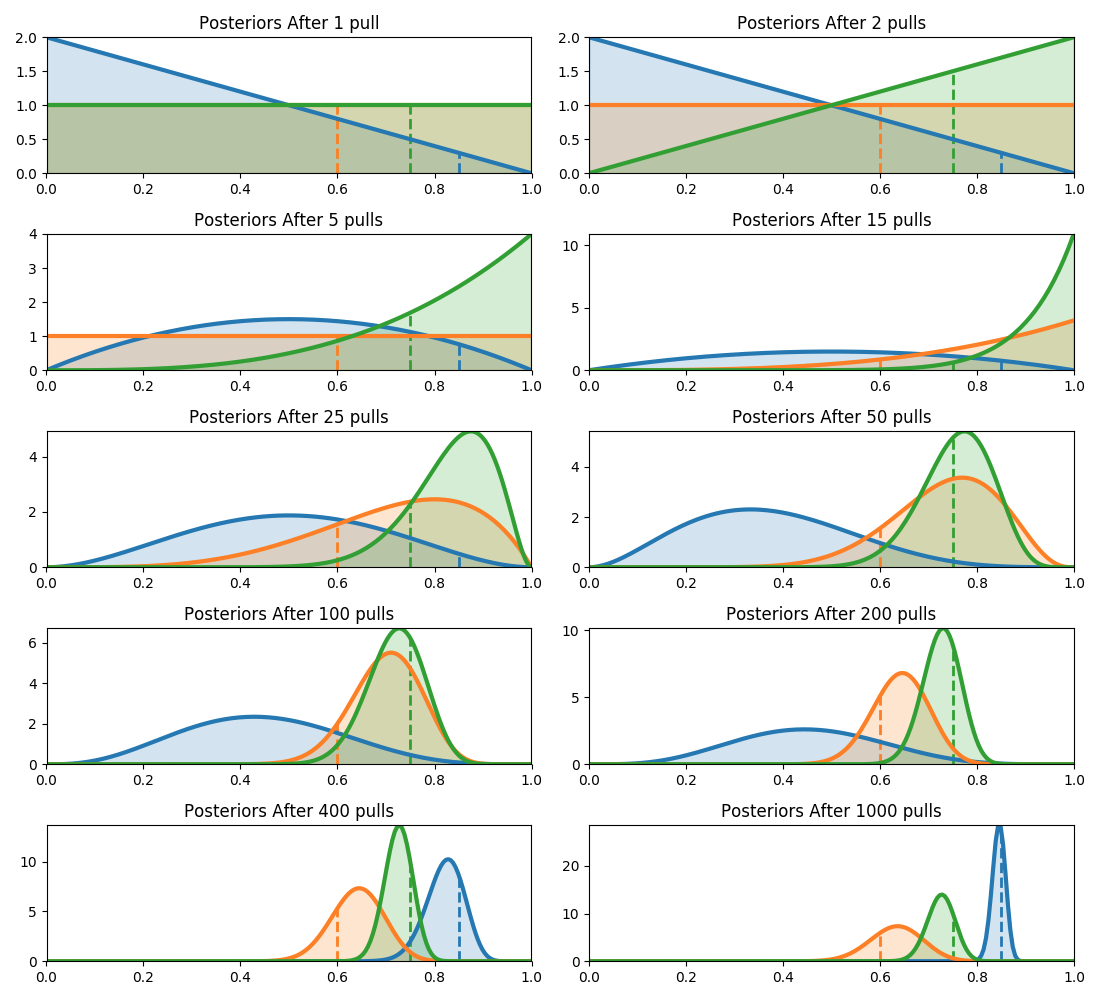### 4. 定义损失函数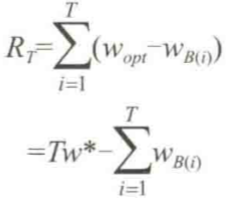### 5. 选择游戏策略

1. 随机：顾名思义，类似于丢色子，这显然不明智，如果用随机策略，就没必要费那么大劲去统计样本以及计算游戏机的后验分布了；
2. 贝叶斯的最大置信边界：选择底层概率的95%置信区间的最大上界的游戏机；
3. 贝叶斯-UCB算法：选择有最大得到的游戏机，其中得分是一个动态的后验分布的分位数；
4. 后验均值：选择具有最大后验均值的游戏机；
5. 最大比例：选择目前观测到的赢的比例最大的游戏机；

# -*- coding: utf-8 -*-

import numpy as np
from IPython.core.pylabtools import figsize
import matplotlib.pyplot as plt
import scipy.stats as stats
import pymc as pm

rand = np.random.rand

figsize(12.5, 5)
from other_strats import *

#define a harder problem
hidden_prob = np.array([0.15, 0.2, 0.1, 0.05])
bandits = Bandits(hidden_prob)

#define regret
def regret(probabilities, choices):
w_opt = probabilities.max()
return (w_opt - probabilities[choices.astype(int)]).cumsum()

#create new strategies
strategies= [upper_credible_choice,
bayesian_bandit_choice,
ucb_bayes ,
max_mean,
random_choice]
algos = []
for strat in strategies:
algos.append(GeneralBanditStrat(bandits, strat))

# train 10000 times
for strat in algos:
strat.sample_bandits(10000)

# test and plot
for i, strat in enumerate(algos):
_regret = regret(hidden_prob, strat.choices)
plt.plot(_regret, label=strategies[i].__name__, lw=3)

plt.title("Total Regret of Bayesian Bandits Strategy vs. Random guessing")
plt.xlabel("Number of pulls")
plt.ylabel("Regret after $n$ pulls");
plt.legend(loc="upper left")

plt.show()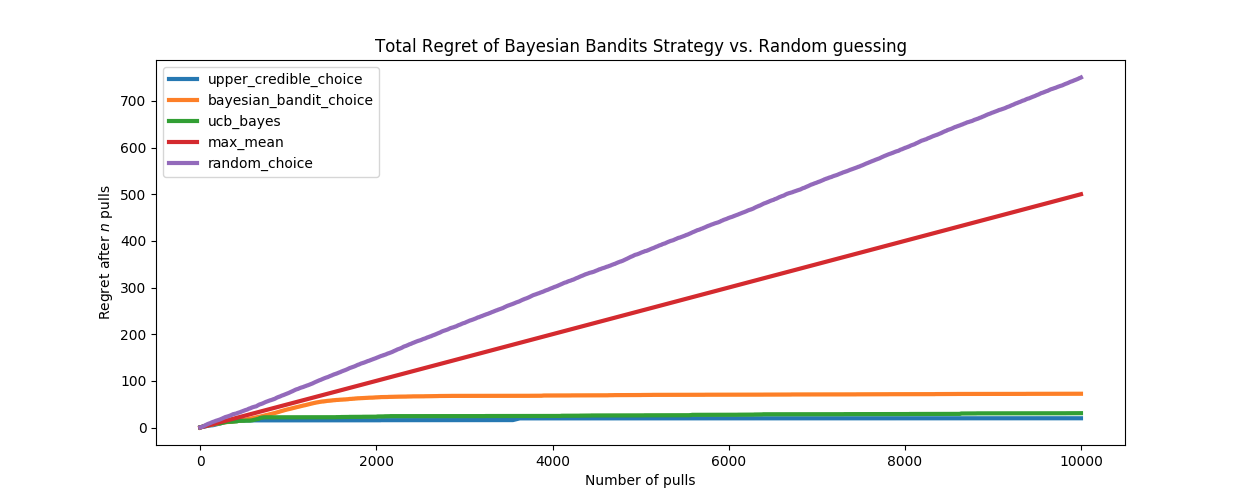### 6. 评估总遗憾期望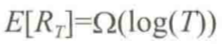# -*- coding: utf-8 -*-

import numpy as np
from IPython.core.pylabtools import figsize
import matplotlib.pyplot as plt
import scipy.stats as stats
import pymc as pm

rand = np.random.rand

figsize(12.5, 5)
from other_strats import *

#define a harder problem
hidden_prob = np.array([0.15, 0.2, 0.1, 0.05])
bandits = Bandits(hidden_prob)

#define regret
def regret(probabilities, choices):
w_opt = probabilities.max()
return (w_opt - probabilities[choices.astype(int)]).cumsum()

#create new strategies
strategies= [upper_credible_choice,
bayesian_bandit_choice,
ucb_bayes ,
max_mean,
random_choice]

trials = 500
expected_total_regret = np.zeros((10000, 3))

for i_strat, strat in enumerate(strategies[:-2]):
for i in range(trials):
general_strat = GeneralBanditStrat(bandits, strat)
general_strat.sample_bandits(10000)
_regret = regret(hidden_prob, general_strat.choices)
expected_total_regret[:, i_strat] += _regret
plt.plot(expected_total_regret[:, i_strat] / trials, lw=3, label=strat.__name__)

[pl1, pl2, pl3] = plt.plot(expected_total_regret[:, [0,1,2]], lw = 3)
plt.xscale("log")
plt.legend([pl1, pl2, pl3],
["Upper Credible Bound", "Bayesian Bandit", "UCB-Bayes"],
loc="upper left")
plt.ylabel("Exepected Total Regret \n after $\log{n}$ pulls");
plt.title( "log-scale of above" );
plt.ylabel("Exepected Total Regret \n after $\log{n}$ pulls");

plt.show()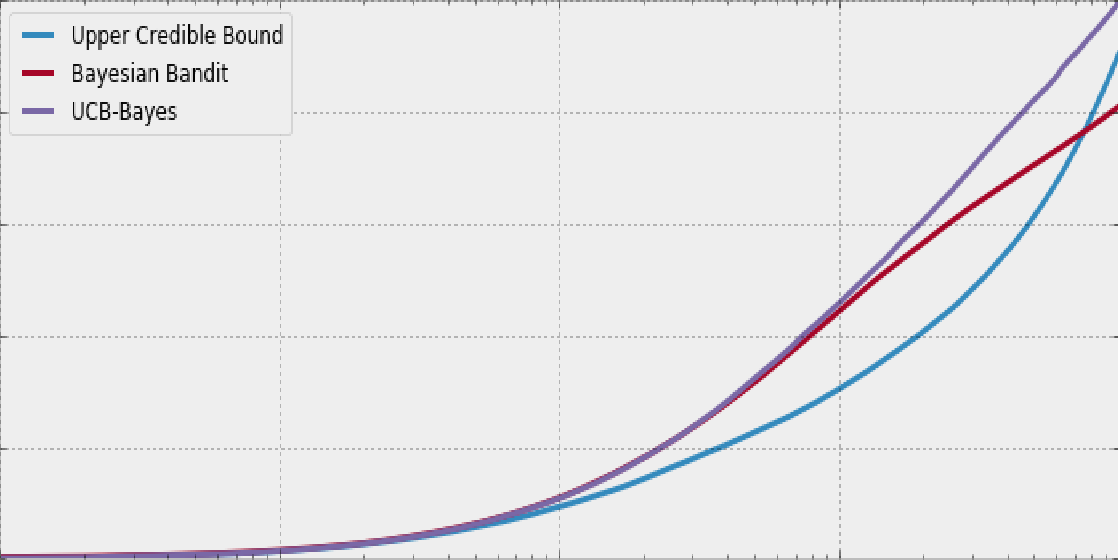## 0x4：算法扩展

### 1. 添加学习速率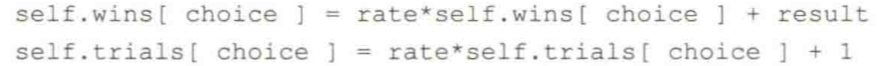1. 如果rate<1，则该算法将更快地忘记先前的获胜，并且会有一个走向无知的下行压力；
2. 如果rate>1，则意味着算法将以风险较高的方式运行，而且更经常地把赌注压在早期赢的游戏机上，对不断变化的环境更有韧性；

### 2. 层次算法

posted @ 2019-02-25 22:02 郑瀚Andrew.Hann 阅读(...) 评论(...) 编辑 收藏